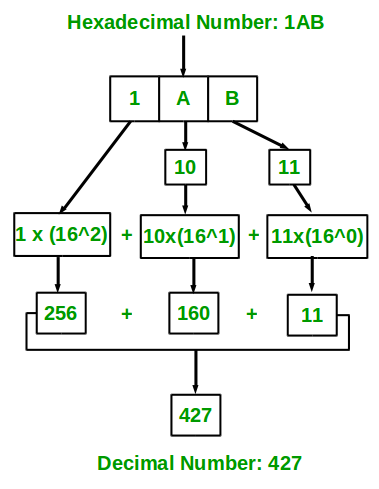Program for hexadecimal to decimal

Given a hexadecimal number as input, we need to write a program to convert the given hexadecimal number into equivalent decimal number.

Examples:

Input : 67
Output : 103

Input : 512
Output : 1298

Input: 123
Output: 291

Recommended: Please try your approach on {IDE} first, before moving on to the solution.

We know that in hexadecimal number uses 16 symbols {0, 1, 2, 4, 5, 6, 7, 8, 9, A, B, C, D, E, F} to represent all numbers. Here, (A, B, C, D, E, F) represents (10, 11, 12, 13, 14, 15).
The idea is to extract the digits of given hexadecimal number starting from right most digit and keep a variable dec_value. At the time of extracting digits from the hexadecimal number, multiply the digit with the proper base (Power of 16) and add it to the variable dec_value. At the end, the variable dec_value will store the required decimal number.

For Example:
If the hexadecimal number is 1A.
dec_value = 1*(16^1) + 10*(16^0) = 26

Below diagram explains how to convert hexadecimal number ( 1AB ) to equivalent decimal value:Below is the implementation of above idea.

C/C++

 // C++ program to convert hexadecimal to decimal #include #include using namespace std;    // Function to convert hexadecimal to decimal int hexadecimalToDecimal(char hexVal[]) {        int len = strlen(hexVal);            // Initializing base value to 1, i.e 16^0     int base = 1;            int dec_val = 0;            // Extracting characters as digits from last character     for (int i=len-1; i>=0; i--)     {            // if character lies in '0'-'9', converting          // it to integral 0-9 by subtracting 48 from         // ASCII value.         if (hexVal[i]>='0' && hexVal[i]<='9')         {             dec_val += (hexVal[i] - 48)*base;                                // incrementing base by power             base = base * 16;         }            // if character lies in 'A'-'F' , converting          // it to integral 10 - 15 by subtracting 55          // from ASCII value         else if (hexVal[i]>='A' && hexVal[i]<='F')         {             dec_val += (hexVal[i] - 55)*base;                        // incrementing base by power             base = base*16;         }     }            return dec_val; }    // Driver program to test above function int main() {     char hexNum[] = "1A";         cout << hexadecimalToDecimal(hexNum) << endl;     return 0; }

Java

 // Java program to convert hexadecimal to decimal import java.io.*;    class GFG  {     // Function to convert hexadecimal to decimal     static int hexadecimalToDecimal(String hexVal)     {            int len = hexVal.length();                 // Initializing base value to 1, i.e 16^0         int base = 1;                 int dec_val = 0;                 // Extracting characters as digits from last character         for (int i=len-1; i>=0; i--)         {                // if character lies in '0'-'9', converting              // it to integral 0-9 by subtracting 48 from             // ASCII value             if (hexVal.charAt(i) >= '0' && hexVal.charAt(i) <= '9')             {                 dec_val += (hexVal.charAt(i) - 48)*base;                                     // incrementing base by power                 base = base * 16;             }                 // if character lies in 'A'-'F' , converting              // it to integral 10 - 15 by subtracting 55              // from ASCII value             else if (hexVal.charAt(i) >= 'A' && hexVal.charAt(i) <= 'F')             {                 dec_val += (hexVal.charAt(i) - 55)*base;                             // incrementing base by power                 base = base*16;             }         }         return dec_val;     }            // driver program     public static void main (String[] args)      {         String hexNum = "1A";             System.out.println(hexadecimalToDecimal(hexNum));     } }    // Contributed by Pramod Kumar

C#

 // C# program to convert  // hexadecimal to decimal using System;    class GFG  { // Function to convert  // hexadecimal to decimal static int hexadecimalToDecimal(String hexVal) {      int len = hexVal.Length;        // Initializing base1 value      // to 1, i.e 16^0     int base1 = 1;        int dec_val = 0;        // Extracting characters as     // digits from last character     for (int i = len - 1; i >= 0; i--)     {          // if character lies in '0'-'9',          // converting it to integral 0-9          // by subtracting 48 from ASCII value         if (hexVal[i] >= '0' &&              hexVal[i] <= '9')         {             dec_val += (hexVal[i] - 48) * base1;                            // incrementing base1 by power             base1 = base1 * 16;         }            // if character lies in 'A'-'F' ,          // converting it to integral          // 10 - 15 by subtracting 55          // from ASCII value         else if (hexVal[i] >= 'A' &&                   hexVal[i] <= 'F')         {             dec_val += (hexVal[i] - 55) * base1;                    // incrementing base1 by power             base1 = base1 * 16;         }     }     return dec_val; }    // Driver Code static void Main()  {     String hexNum = "1A";      Console.WriteLine(hexadecimalToDecimal(hexNum)); } }    // This code is contributed by mits

PHP

 = 0; \$i--)     {          // if character lies in '0'-'9',          // converting it to integral 0-9          // by subtracting 48 from ASCII value.         if (\$hexVal[\$i] >= '0' &&              \$hexVal[\$i] <= '9')         {             \$dec_val += (ord(\$hexVal[\$i]) - 48) *                                           \$base;                                // incrementing base by power             \$base = \$base * 16;         }            // if character lies in 'A'-'F' ,           // converting it to integral          // 10 - 15 by subtracting 55          // from ASCII value         else if (\$hexVal[\$i] >= 'A' &&                   \$hexVal[\$i] <= 'F')         {             \$dec_val += (ord(\$hexVal[\$i]) - 55) *                                           \$base;                        // incrementing base by power             \$base = \$base * 16;         }     }            return \$dec_val; }    // Driver Code \$hexNum = "1A";  echo hexadecimalToDecimal(\$hexNum);    // This code is contributed by mits ?>

Output:

26

This article is contributed by Harsh Agarwal. If you like GeeksforGeeks and would like to contribute, you can also write an article using contribute.geeksforgeeks.org or mail your article to contribute@geeksforgeeks.org. See your article appearing on the GeeksforGeeks main page and help other Geeks.

Please write comments if you find anything incorrect, or you want to share more information about the topic discussed above.My Personal Notes arrow_drop_up

Improved By : Mithun Kumar, soumya7

1

Please write to us at contribute@geeksforgeeks.org to report any issue with the above content.